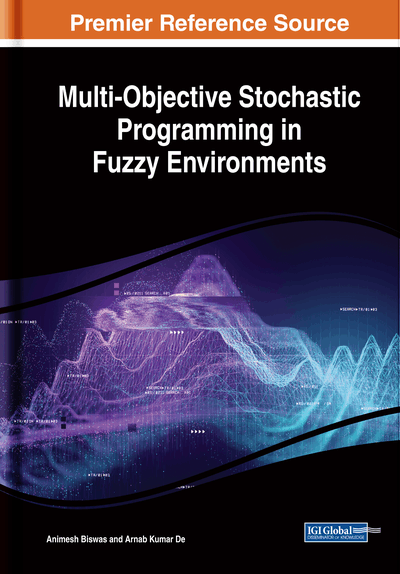# Fundamental Concepts

DOI: 10.4018/978-1-5225-8301-1.ch002
Available
\$33.75
List Price: \$37.50
10% Discount:-\$3.75
TOTAL SAVINGS: \$3.75

## Abstract

In this chapter, the authors discuss some basic concepts of probability theory and possibility theory that are useful when reading the subsequent chapters of this book. The multi-objective fuzzy stochastic programming models developed in this book are based on the concepts of advanced topics in fuzzy set theory and fuzzy random variables (FRVs). Therefore, for better understanding of these advanced areas, the authors at first presented some basic ideas of probability theory and probability density functions of different continuous probability distributions. Afterwards, the necessity of the introduction of the concept of fuzzy set theory, some important terms related to fuzzy set theory are discussed. Different defuzzification methodologies of fuzzy numbers (FNs) that are useful in solving the mathematical models in imprecisely defined decision-making environments are explored. The concept of using FRVs in decision-making contexts is defined. Finally, the development of different forms of fuzzy goal programming (FGP) techniques for solving multi-objective decision-making (MODM) problems is underlined.

## Complete Chapter List

Search this Book:
Reset